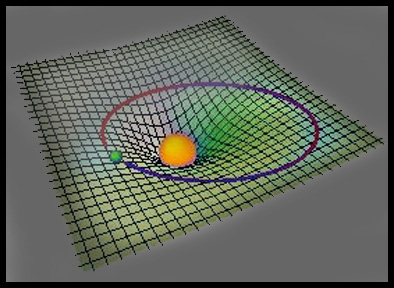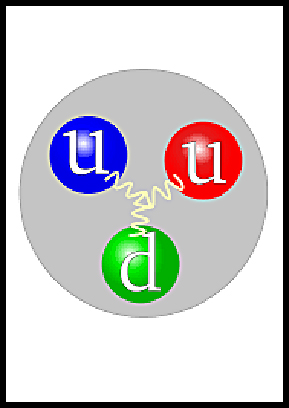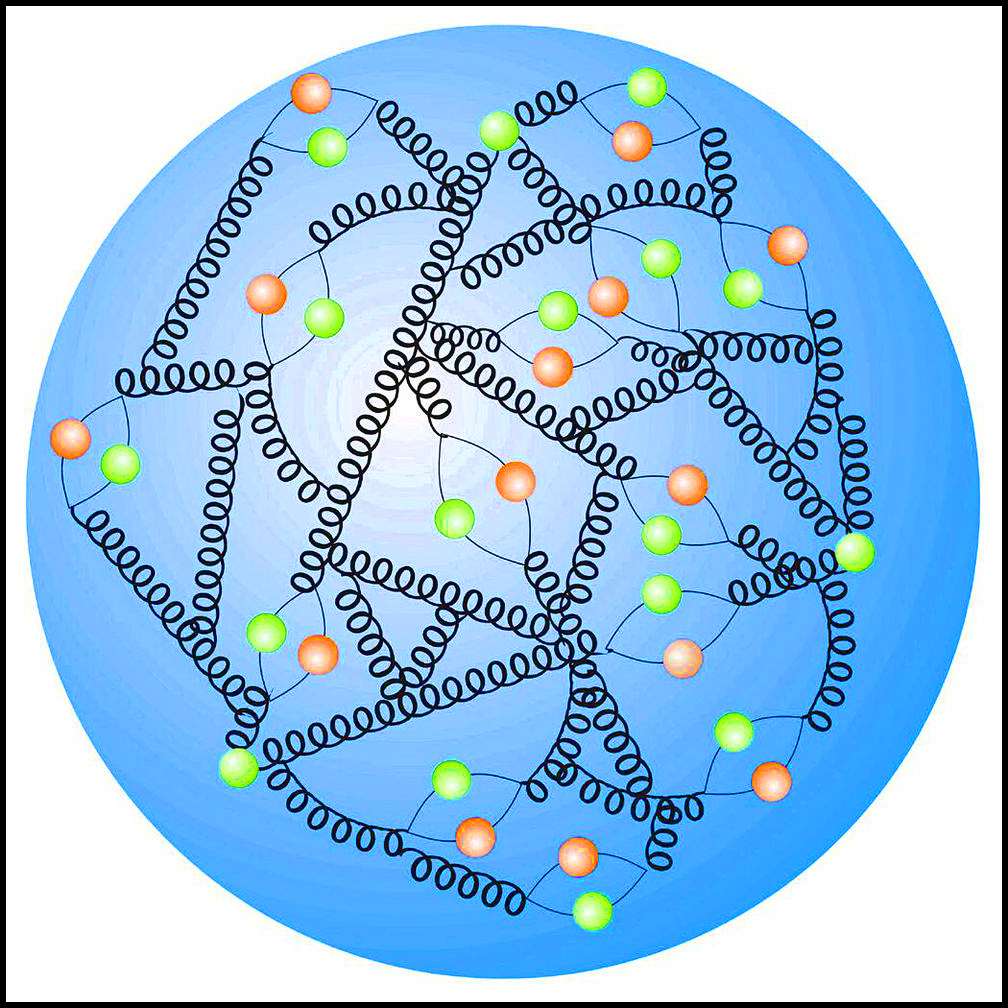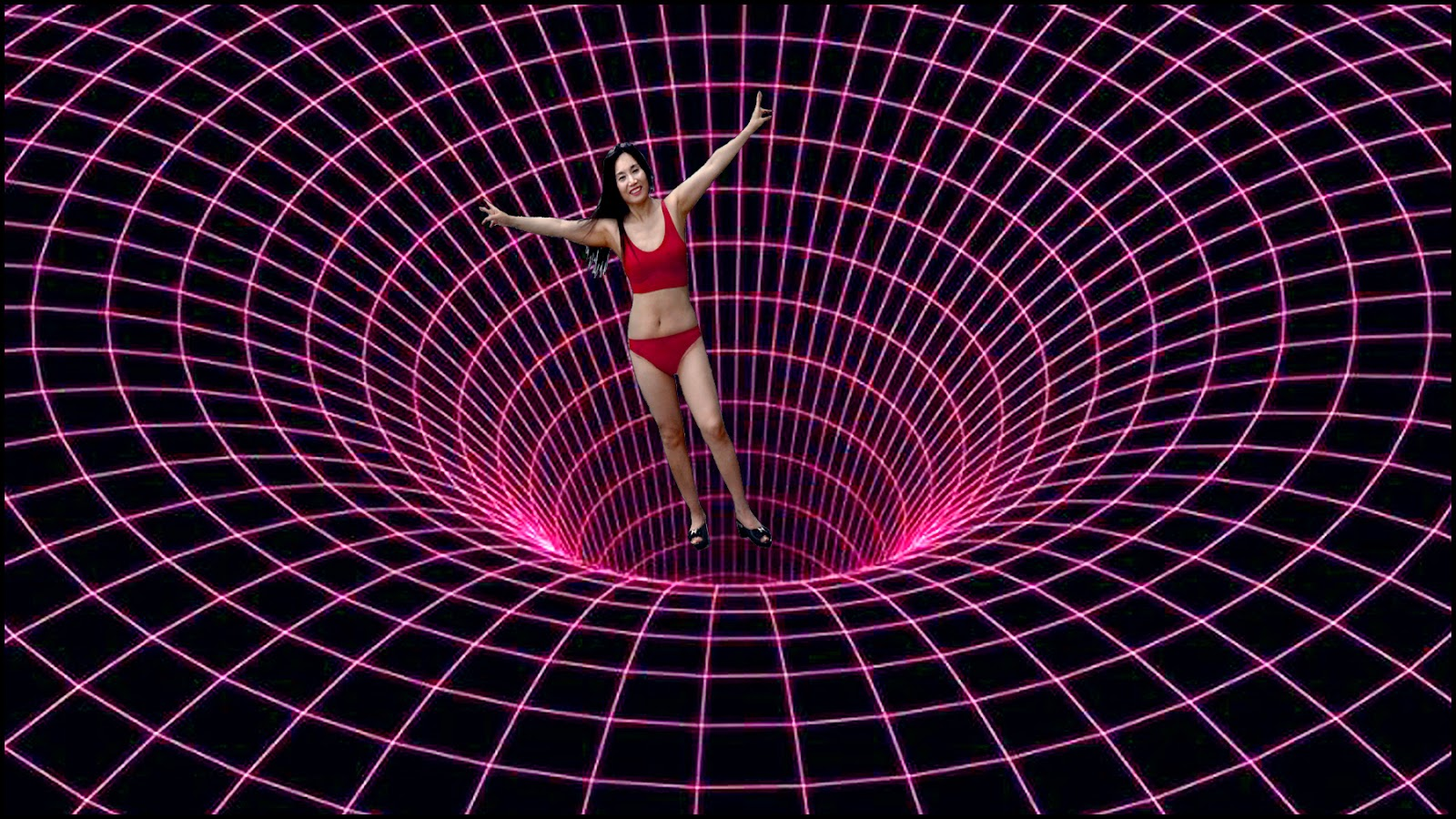Gravity is the weakest of the 4 forces, namely the weak nuclear force, the strong nuclear force and the electromagnetic force. Gravity wants to attract more matter to itself. But, normal gravitational attraction between 2 protons is so small that it is unmeasurable. Gravity can safely be ignored by chemists when they study how groups of atoms bond together to form molecules. But what’s the real truth about gravity?We have all experienced the pain of gravity when we have fallen down.

Nonetheless, gravity is the dominant force in real life. It holds us to the ground and keeps the moon and planets in their orbits. Gravity is always an attraction force much like the Law of Attraction, gravity is very important in the universe.

The only force that we experience every second of every day is the force of Gravity. We are so accustomed to gravity that when it’s not present we are disoriented. I remember falling into a hole at work and feeling no sensation of falling until my face hit the ground.

Time is how we experience gravity. The gravitational force on Earth is measured as g= 9.8 meters per second per second. Since, time is related to the speed of light then gravity is also related to time.

Is Gravity a force and an energy? Perhaps...

## Gravity inside Protons and Neutrons

Inside atoms the Newtonian energy of gravity between particles doesn’t exist! Instead, the Quantum force of Gravity is born!

The strong nuclear force that holds quark particles together comes from the gluon binding force. This force acts in two ways, first it holds quarks together to create protons and neutrons and it also creates a force called gravity. Wait a minute, let me explain below...

But first consider that, electric charges can repel each other as well as attract. Any object  has a huge amount of atoms made up of a positively charged nucleus surrounded by negative electrons but the positive and negative charges cancel each other out.

However, everything that has mass, has the same gravitational attraction ‘force’. So gravity becomes an additive and stronger force relative to electrical or nuclear forces in much  larger objects.

Note that objects have a mass but gravity gives the object its weight. The more the mass the stronger the attraction to the Earth. In space you are weightless because you are far away from the Earth but you are not massless.

# Can Gravity Bend Space?This drawing shows that the force field of gravity is stronger the closer the objects are to each other, also gravity is a long range force that extends the energy field as in this sketch.

## Einstein, can gravity bend space?

My question is how can gravity bend space? Gravity is a mass attracting mass force so how would it bend space which has almost nothing in it and is almost a total vacuum?

Einstein tried to understand gravity as the curvature of space instead of a force that attracts more mass to itself. He came up with this (out of the world) theory because he believed that light was massless.

His theory allowed him to explain why light was attracted to gravity. The easy way to prove Einstein wrong is to prove light has mass. But what about using logic?

## Source of Gravity

What is the force of gravity inside large objects such as stars and black holes? Remember the property of gravity is an attraction between objects. It wants to attract more matter to itself.

Scientists have finally looked inside the atom and found it contains quarks held together by gluons and orbitals of electron particles. A proton is made up of 3 quarks which has a total electric charge of plus one that balances the electron’s minus one charge.

Quarks in a proton are held together not by an electrical force or a unknown force but by gluons which are kind of like a glue called the strong nuclear force.Gluons are strange because the farther apart the quarks move from each other the stronger the glue force becomes. Thus preventing the protons from pulling apart due to the repulsive force of positive electric charges of the 3 quarks..

## Strong Nuclear force creates Gravity

If each small proton and neutron has this energy this is what gravity is made of. The gluon is an attractive binding force to other gluons just like the attractive force of gravity.

All particles of matter are made up of atoms that are constantly vibrating and the hotter the temperature the faster they vibrate. Any extra heat energy that is added to a particle and you find it has more mass.

This mass is due to the extra energy that the gluons are producing in order to keep the quarks together.

The observed result is that the mass of atoms is about 99% from other sources such as the gluon energy and not from the rest mass of the particles.

There is no doubt that gravity is a property of mass! Space doesn’t curve itself to attract matter. Matter must first arrive to create stars and Black Holes. Did Einstein put the cart before the horse?

The vibration of quark particles in atoms creates a need for a strong force and this gluon force field must be the source of gravity...

The fact that the gluon force is stronger during vibrational molecular motion when the quarks are farther apart, means it is a long range force like gravity.

# Gluons and Quarks during the Big Bang

When temperatures reach into millions of degrees the gluon force breaks down into a plasma of quarks and gluons as at the time of the Big Bang.

What if gravity wasn’t a force until a few thousand thousands of a second later?

They say that during an Electroweak Epoch from 10-36 seconds to 10–12 seconds the gluons were separate from the other two forces. The field of particles was more like a field of radiation having very little mass.

This allowed the particles to move faster than the speed of light into a void of space to become a homogenous universe into a uniform soup of plasma.

Eventually the soup cooled down and the Higgs field added some mass to the Big bang reaction.

Shortly after gluons added mass to protons and atoms as the universe became full of matter containing  mass and gravity.In this molecule drawing notice the many spring like gluons holding quarks together. Is this the source of Gravity?

## Where did Gravity come from?

During the big bang photon radiation entered the nothingness of empty space and the Universe was created.

Try to imagine nothingness which has no space or time. When the light from source created the 3rd dimension it brought time with it.

The speed of light is invariant (unchanging) and the speed has time as the only constant of light.

That means that time is also invariant or unchanging. Light doesn’t move backwards or stand still. It’s always moving forward.

It’s amazing that the light changed to matter and in the matter was the same quality of time in the vibrations of matter and the force of gravity.

## The Gravitaional Constant ‘G’

Isaac Newton’s law of gravity is given by the formula

F (force) = G x m1 x m2 /r^2

As it turns out the Gravitational constant G is invariant and is the value of G = 6.674215 x 10^-11m^3 /Kg/sec/sec. Accurate to 46ppm. Note that time is a constant in the value of G.

A kilogram mass has the same force of gravity (g) on earth or in space because the mass doesn’t change. Gravity is a force and on earth the amount is g(earth) which equals 9.8 meters per second per second.

On the moon a kilogram mass weighs less but it’s force of gravity is the same only the gravitational force of g(moon) is less. On jupiter the force of g(Jupiter) would be stronger because the kilogram mass is reacting with the larger mass of Jupiter.

The kilogram mass remains the same and the amount of mass governs the force of gravity.

## Quantum effects of Gravity in Special cases

At the quantum level of particles the force of gravity can vary depending of the speed of the matter. This change is predicted by Einstein’s theory of relativity.

When matter travels faster its mass will increase and its size decreases.

Accurate atomic clocks have been used to prove that time ticks slower for a moving clock (matter).  This is known as time dilation and has led to all kinds of science fiction involving time travel.

## Gravity and Time Dilation

The false assumptions made are that time changes and that time is not invariant. The speed of light and time are constant and unchanging. Therefore, what is the cause that makes the clocks tick slower?

The truth is that only the properties of matter change. The quantum particles increase in mass due to the extra speed. Relativity adds extra mass produced by the quarks and gluons.

Because of the extra mass the force of gravity in molecules increases slowing down the atomic vibrations causing the clocks to tick slower. But time itself is invariant and constant.

The Ultimate Truth is that time itself doesn’t change. Therefore, time travel is science fiction and impossible. The Twin Paradox is a hoax!In the image a girl falls toward a field of gravity. If this was a black hole the immense gravity would rip you apart instantly.

## GPS system and Time

The 24 satellites in orbit for the GPS system provide accurate location and time for cell phone users. The time must be adjusted daily due to the quantum changes in matter.

As with the increase in speed discussed earlier the time ticks slower than the clocks on earth. However, the time ticks faster due to less gravity.

Because the satellites are orbiting about 20,000 km they are far away from the earth’s gravity field. The smaller force of gravity allows the clocks to tick faster than the clocks on earth.

Thus the satellite clocks run 38 microseconds faster than earth clocks.

The false assumption is that a clock falling into a black hole will seem to slow down and stop time. Since this is impossible, the reason time slows is due to the massive gravity in a black hole.

The truth is that time itself is constant but clocks would slow down due to the massive gravity acting on molecules. Einstein’s spacetime is based on falsehoods.

### Key Takeaways

1. We experience the gravitational force of attraction every second of our life

2. Gravity is an invariant force dependent on mass and the constant of time.

3. The source of gravity is in the quantum fluctuations of quarks and gluons

4. Time and the gravitational constant (G) are invariant

5. Quantum effects such as speed and gravity effects only affect physical matter

6. Time travel are fictional ideas

7. Spacetime can not be stretched or shortened to explain gravity

If you resonate with this new information I want you to be on my tribe of followers. I would like to encourage your dreams and confirm your thoughts that science of light will show us the Ultimate Truth. I care about you and hope you will join this journey into understanding science and light.

Your influence is greater than you can imagine!

Join my list of subscribers and get my latest post in your email.

Thanks for being here. As always, be well.

https://lovinthings.com

### About the Author Erik Lovin

Erik has a BSc degree and is a retired professional photographer who is now a published Author of many books. His passion is understanding how life and the universe works. He is currently blogging about the science of the Big Bang and the science of cosmology. Erik is helping his tribe with questions about the universe. His goal is to help find a theory of everything (TOE). In order to do that, he is trying to prove light has mass and that the fabric of spacetime is a false theory. We are welcoming questions and answers that you might have about the universe.